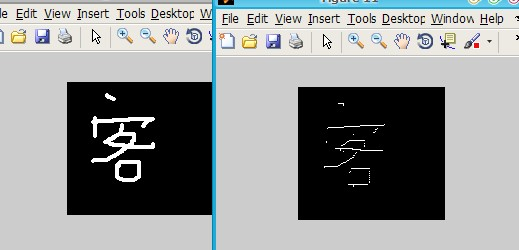# 圖像細化（骨架化）算法 分析

【    p9    p2     p3

p8    p1     p4

p7    p6     p5 】

（其中p1為白點，如果以下四個條件同時滿足，則刪除p1，即令p1=0）

過程1 細化刪除條件為：        （1）、2 < =N(p1) <= 6，   N(x)為x的8鄰域中黑點的數目

（2）、A(p1)=1,    A(x)指的是將p2-p8之間按序前後分別為0、1的對數（背景色：0

（3）、p2*p4*p6=0

（4）、p4*p6*p8=0

如果同時滿足以上四個條件則該點可以刪除（賦值為0）。

過程2 細化刪除條件為：        （1）、2 < =N(p1) <= 6，   N(x)為x的8鄰域中黑點的數目

（2）、A(p1)=1,    A(x)指的是將p2-p8之間按序前後分別為0、1的對數（背景色：0

（3）、p2*p4*p8=0

（4）、p2*p6*p8=0

如果同時滿足以上四個條件則該點可以刪除。

A.m

1 function n=A(temp,i,j)2 %0->1的數目3 shuzu=[temp(i,j),temp(i-1,j),temp(i-1,j+1),temp(i,j+1),temp(i+1,j+1),temp(i+1,j),temp(i+1,j-1),temp(i,j-1),temp(i-1,j-1)];4 n=0;5 for i=2:86     if shuzu(i)==0&&shuzu(i+1)==17         n=n+1;8     end9 end

 1 test=input('Please input a digits image:','s'); %輸入圖像 2 x=imread(test); 3 if ~isbw(x) 4     '請確保輸入圖像為二值化圖像！'; 5 else  6     [height,width]=size(x); 7     mark=1; 8 %     temp=zeros(height+2,width+2); 9 %     temp(2:height+1,2:width+1)=x(:,:);10     temp=x;11     imshow(temp);12    while mark==113     mark=0;14         15         for i=2:height-116             for j=2:width-117                 condition=0;18              %判斷P(r,c)是否為可細化像素19                if temp(i,j)==120                  n=0;21                  for ii=-1:122                      for jj=-1:123                          n=n+temp(i+ii,j+jj);24                      end25                  end26              if (n>=3 && n<=7)27                  condition=condition+1;28              end 29              if A(temp,i,j)==130                  condition=condition+1;31              end32              if temp(i-1,j)*temp(i,j+1)*temp(i+1,j)==033                 condition=condition+1;34             end35             if temp(i,j+1)*temp(i+1,j)*temp(i,j-1)==036                 condition=condition+1;37             end38           if condition==4           39                mark=1;40                temp(i,j)=0;41           end42                end43             end44         end45         figure;imshow(temp);46         47         48         for i=2:height-149             for j=2:width-150                 condition=0;51              %判斷P(r,c)是否為可細化像素52                if temp(i,j)==153                  n=0;54                  for ii=-1:155                      for jj=-1:156                          n=n+temp(i+ii,j+jj);57                      end58                  end59              if (n>=3 && n<=7)60                  condition=condition+1;61              end 62              if A(temp,i,j)==163                  condition=condition+1;64              end65              if temp(i-1,j)*temp(i,j+1)*temp(i,j-1)==066                 condition=condition+1;67             end68             if temp(i,j-1)*temp(i+1,j)*temp(i,j-1)==069                 condition=condition+1;70             end71           if condition==4           72                mark=1;73                temp(i,j)=0;74           end75                end76             end77         end78         figure;imshow(temp);79     end  80 end